# How to Combine Text and Formatted Numbers into a Single Entity in Excel in Excel

In this article, we will learn How to Combine Text and Formatted Numbers into a Single Entity in Excel in Excel.

Scenario:

Excel considers number values in many formats like number, date, time, text, percentage or currency. These can be changed into each other. Excel converts numbers to text to use numbers as text in formulas like vlookup with numbers and text. The Excel TEXT function lets you convert the number to text. The TEXT function in Excel is used to convert numbers into text. The fun part is you can format that number to show it in the desired format. For example format a yy-mm-dd date into dd-mm-yy format. Add currency signs before a  number and many more.

TEXT formula in Excel

TEXT function is a string function which converts any value to a given format. The result may seem that it is a number but it's in text format.

 =TEXT(cell_ref, text_format)

cell_ref : value to convert using cell reference

Text_format : Format to convert

 Format Output_format d 7 ddd Thu dddd Thursday m 2 mmm Feb mmmm February mmmmm F yy 19 yyyy 2019 m/d/y 2/7/19 mm/dd/yy 02/07/19 mm/dd/yyyy 02/07/2019 ddd, mmm d Thu, Feb 7 mm/dd/yyyy h:mm AM/PM 02/07/2019 2:15 PM mm/dd/yyyy hh:mm:ss 07/02/2019 14:15:00

Example :

All of these might be confusing to understand. Let's understand how to use the function using an example. Here we have some examples to convert date values to text format or any other required format.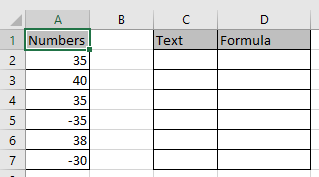Convert the value in A2 cell.

Use the formula in C2 cell

 =TEXT (A2, “0”)

“0”: returns the general text format.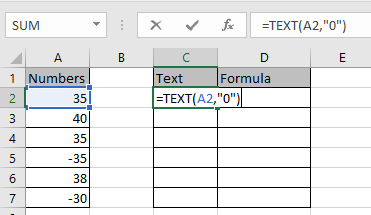Press Enter and copy the formula in remaining cells using Ctrl + D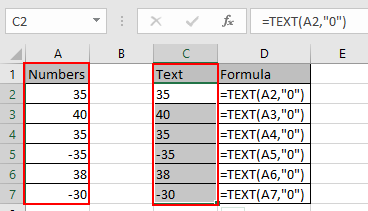As you can see we got numbers as text output because the significance number always varied.

Here we have some numbers to convert to text format or any other required format.

Use the formula:

 =TEXT(A2,"d")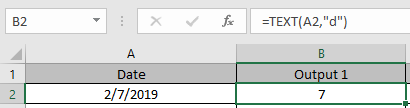As you can see the value in the output cell is in Text format.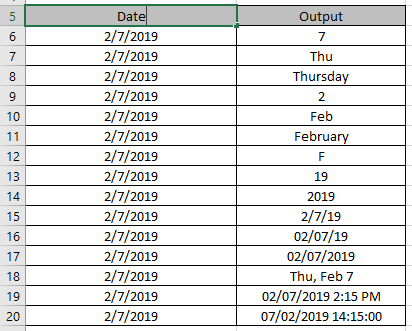You can use any Format_text and do your work in excel without any interruption

# Add 0 before a Number

Sometimes you are needed to add 0 before some fixed digit of numbers like phone number or pin number. Use this Text formula to do so…

 =TEXT( 1234, “00000”)

If you have N digits of number then in text format argument write n+1 0s.

# Add Currency Before a Number

Write this text formula to add currency.

 =TEXT( 1234, “\$0”)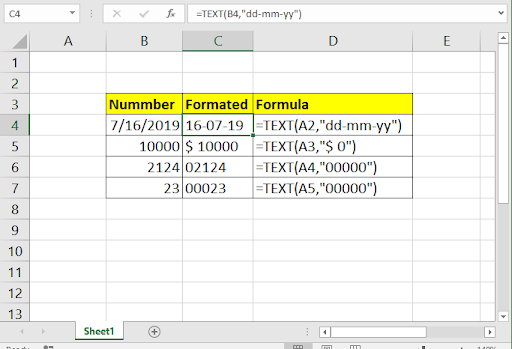As you can see we got numbers as text output because the significance number always varied.

Concatenating a text string and a formula-calculated value. To make the result returned by some formula more understandable for your users, you can concatenate it with a text string that explains what the value actually is.
For example, you can use the following formula to return the current date:

 =CONCATENATE("Today is ",TEXT(TODAY(), "dd-mmm-yy"))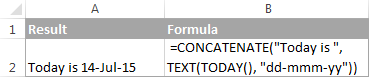Using CONCATENATE in Excel - things to remember

To ensure that your CONCATENATE formulas always deliver the correct results, remember the following simple rules:

Excel CONCATENATE function requires at least one "text" argument to work.

Here are all the observational notes using the formula in Excel
Notes :

1. Use & operator to combine text. & operator does the same work as CONCAT function
2. In new versions of Excel, CONCATENATE is replaced with the CONCAT function, which has exactly the same syntax. The CONCATENATE function is kept for backward compatibility, it is common practice to use CONCAT instead because Excel does not give any promises that CONCATENATE will be available in future versions of Excel.

Hope this article about How to Combine Text and Formatted Numbers into a Single Entity in Excel in Excel is explanatory. Find more articles on calculating values and related Excel formulas here. If you liked our blogs, share them with your friends on Facebook. And also you can follow us on Twitter and Facebook. We would love to hear from you, do let us know how we can improve, complement or innovate our work and make it better for you. Write to us at info@exceltip.com.

Related Articles :

Excel REPLACE vs SUBSTITUTE function: The REPLACE and SUBSTITUTE functions are the most misunderstood functions. To find and replace a given text we use the SUBSTITUTE function. Where REPLACE is used to replace a number of characters in a string.

How to use the ISTEXT function in Excel : returns the TRUE logic value if the cell value is text using the ISTEXT function in Excel.

How to Highlight cells that contain specific text in Excel : Highlight cells based on the formula to find the specific text value within the cell in Excel.

Converts decimal Seconds into time format : As we know that time in excel is treated as numbers. Hours, Minutes, and Seconds are treated as decimal numbers. So when we have seconds as numbers, how do we convert into time format? This article got it covered.

Calculate Minutes Between Dates & Time in Excel : calculating the time difference is quite easy. Just need to subtract the start time from the end time. Learn more about this formula clicking the link

Replace text from end of a string starting from variable position : To replace text from the end of the string, we use the REPLACE function. The REPLACE function use the position of text in the string to replace.

Popular Articles :